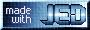# Loving ISIS - Confessions of a Former XSPEC User

Models

Being very used to the XSPEC `model` command, I have aliased (but otherwise have in no way redefined) the ISIS `fit_fun();` command to `model();`. I will use this latter syntax throughout.

There are several differences between how ISIS and XSPEC interpret models. Perhaps the most important of these is that ISIS uses `S-lang` to parse the model expression, and as far as `S-lang` is concerned, ISIS models are just vector functions. An important corollary of this is: Most 'mathematically sensible' ISIS model expressions will evaluate pretty much the way you expect them to, when the equivalent expression would fail in XSPEC. As an example, compare the following two model definitions:

```     isis> model("(constant(1)-edge(1))*phabs(1)*(powerlaw(1)+gaussian(1))");

xspec> model (constant-edge)*phabs*(powerlaw+gauss)
```
The ISIS model expression is perfectly valid (and does what you expect it would), whereas the XSPEC expression won't even parse. ISIS allows for a real increase in the economy of complicated model expressions compared to the functional equivalents in XSPEC.

There are a number of important points to notice in the above example.

• Unlike XSPEC, ISIS does not allow model names to be abbreviated. (Just as in Sherpa, however, their names can be aliased; e.g., `alias_fun("gaussian","line");`).
• ISIS models are string expressions in quotes.
• All models have a numerical identifier. (If not input, the identifier defaults to `1`.)
• If properly built (see the ISIS manual), ISIS provides access to all XSPEC models, including local models, using their familiar names. The one exception to this rule is that the XSPEC `gauss` model is renamed to the ISIS `gaussian` model. (In ISIS, `gauss()` refers to a gaussian defined in wavelength space.)
The numerical model identifier can be used in a number of useful ways. First, it allows multiple instances of the same model. For example:
```     isis> model("powerlaw(1)+powerlaw(2)");
```
Once a model's parameters are set (and/or fit), the default will be for that numerically identified instance of a model to use its last set of valid parameters. That is, if you define a model and parameters, define a subsequent model, then define a third model that uses components from the first model definition, the model parameters will carry over from the first definition. As an example:
```     isis> model("powerlaw(1)+powerlaw(2)");
isis> edit_par;
isis> () = fit_counts;
isis> model("powerlaw(1)+powerlaw(2)+powerlaw(3)");
```
We define a two powerlaw model and then edit the parameters. `edit_par` will pull up whatever you use as your default editor, and let you edit the parameter values by hand. (I find this much easier than the XSPEC line-by-line parameter editing.) We then fit the model, decide it needs another component, so redefine the model with whatever extra components we need. The previously fitted parameters are retained. I.e., we do not need the XSPEC `addmod` command.

The second way the numerical identifier can be used is to allow for different model instances to be defined for different datasets via the `Isis_Active_Dataset` variable. In the example analysis script, the model fits a different normalization constant to the PCA and HEXTE data in this manner. This is in fact a very common procedure in X-ray astronomy. We can define a model as such:

```     isis> model("constant(Isis_Active_Dataset)*(powerlaw(1))");
```
The same `powerlaw` will be applied to each dataset, but the `constant` model will be different for each. This brings up another thing I prefer in ISIS over XSPEC: ISIS presumes everything is the same for each dataset, until you tell it differently. If in the above you have five simultaneous datasets, `edit_par;`, `list_par;` or `list_free;` will show you only one set of `powerlaw` parameters, but five sets of `constant` parameters. Again, there is a greater economy in the ISIS syntax.

The third way the numerical identifier can be used, with the `Isis_Active_Dataset` variable, is shown in this example:

```     isis> define line ( )
{
switch (Isis_Active_Dataset)
{case 1 or case 3: return gaussian(1);}
{case 2 or case 4: return lorentz(1);}
{return diskline(1);}
}

isis> model("phabs(1 )*( powerlaw(1) + line( ) )");
```
I.e., we have defined a model, `line()`, that when invoked, e.g.: `isis> model("powerlaw(1)+line()");` will return different kinds of line models for different datasets. (I haven't quite found a use for this yet, but it is cool.)

A further important difference between ISIS and XSPEC alluded to above is that ISIS does not distinguish between "additive" and "multiplicative" models. In the examples above, the `constant` model is used multiplicatively, whereas for the power spectrum example shown here, the `constant` model was used additively (to model the white noise level). That's because ISIS only cares that the model is a vector of numbers, not how the numbers are being used. This allows you to do a lot of powerful things. (It also allows you to do a lot of stupid things, but, with great power ...)

The only kind of model that ISIS does treat differently is a convolution model. Its syntax can be seen in the following example:

```     isis> model("reflect(1, phabs(1)*(powerlaw(1)+gaussian(1)))");

```
As long as you follow the syntax of: ``` model( "convolution(id, expression )"); ```, everything should be fine.

Next up: Parameters.

This page was last updated Oct 21, 2020 by Michael Nowak. To comment on it or the material presented here, send email to mnowak@physics.wustl.edu.MIT Accessibility